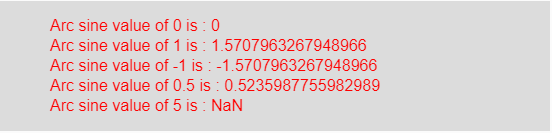# p5.js | asin() function

• Last Updated : 10 Apr, 2019

The asin() function in p5.js is used to calculate the inverse of sine (arc sine). If the input value range is -1 to 1 then it returns the range between -π/2 to π/2.

Syntax:

Hey geek! The constant emerging technologies in the world of web development always keeps the excitement for this subject through the roof. But before you tackle the big projects, we suggest you start by learning the basics. Kickstart your web development journey by learning JS concepts with our JavaScript Course. Now at it's lowest price ever!

`asin(value)`

Parameters: This function accepts a single parameter value which stores the domain of asin() function.

Return Value: It returns the arc sine of given value.

Below program illustrates the asin() function in p5.js:

Example: This example uses asin() function to get arc sine of a value.

 `function` `setup() { ``  ` `    ``// Create Canvas of given size``    ``createCanvas(550, 130); ``} ``  ` `function` `draw() { ``      ` `    ``// Set the background color ``    ``background(220); ``      ` `    ``// Initialize the parameter``    ``// with some values``    ``let a = 0; ``    ``let b = 1; ``    ``let c = -1;``    ``let d = 0.5;``    ``let e = 5;``      ` `    ``// Call to asin() function ``    ``let v = asin(a);``    ``let w = asin(b);``    ``let x = asin(c);``    ``let y = asin(d);``    ``let z = asin(e);``      ` `    ``// Set the size of text ``    ``textSize(16); ``      ` `    ``// Set the text color ``    ``fill(color(``'red'``)); ``    ` `    ``// Getting arc sine value ``    ``text(``"Arc sine value of 0 is : "` `+ v, 50, 30);``    ``text(``"Arc sine value of 1 is : "` `+ w, 50, 50);``    ``text(``"Arc sine value of -1 is : "` `+ x, 50, 70);``    ``text(``"Arc sine value of 0.5 is : "` `+ y, 50, 90);``    ``text(``"Arc sine value of 5 is : "` `+ z, 50, 110);     ``} `

Output:Note: If the value is greater than 1 or less than -1 then it returns NaN.

Reference: https://p5js.org/reference/#/p5/asin

My Personal Notes arrow_drop_up